Last month, @freakonometrics posted a bunch of articles on his blog about the North pole (in French):

There are some maps on these articles that were produced using ggplot2 excellent package in R. We want to share our code here.

First, let’s get a world map. We’ll use the getMap function from the rworldmap package. I prefer this function to the map one, because it gives more accurate and more up to date borders. I used the same method when I explained how to draw the European Union map.

library(rworldmap)
library(dplyr)
library(ggplot2)
library(geosphere)
library(gpclib)

# World map
worldMap <- getMap()
world.points <- fortify(worldMap)
world.points$region <- world.points$id

world.df <- world.points[,c("long","lat","group", "region")]

worldmap <- ggplot() +
geom_polygon(data = world.df, aes(x = long, y = lat, group = group)) +
scale_y_continuous(breaks = (-2:2) * 30) +
scale_x_continuous(breaks = (-4:4) * 45)

worldmap


That code gives the following map: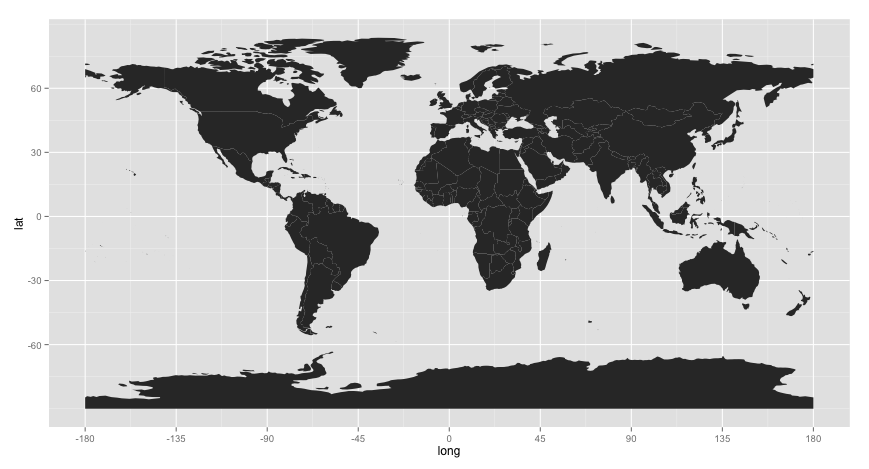World map with ggplot2

There is an awesome function that enables us to change the projection for the map in a split second: coord_map, still in ggplot package. Playing with the orientation is easy too.

worldmap <- ggplot() +
geom_polygon(data = world.df, aes(x = long, y = lat, group = group)) +
scale_y_continuous(breaks = (-2:2) * 30) +
scale_x_continuous(breaks = (-4:4) * 45) +
coord_map("ortho", orientation=c(61, 90, 0))
worldmap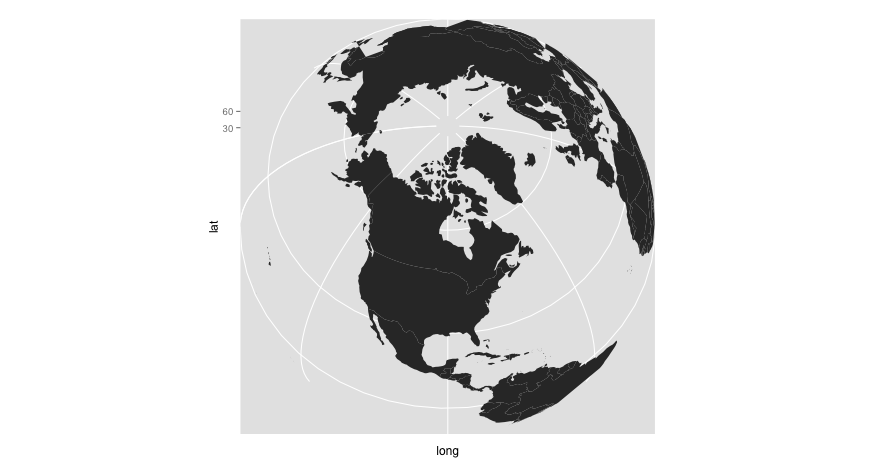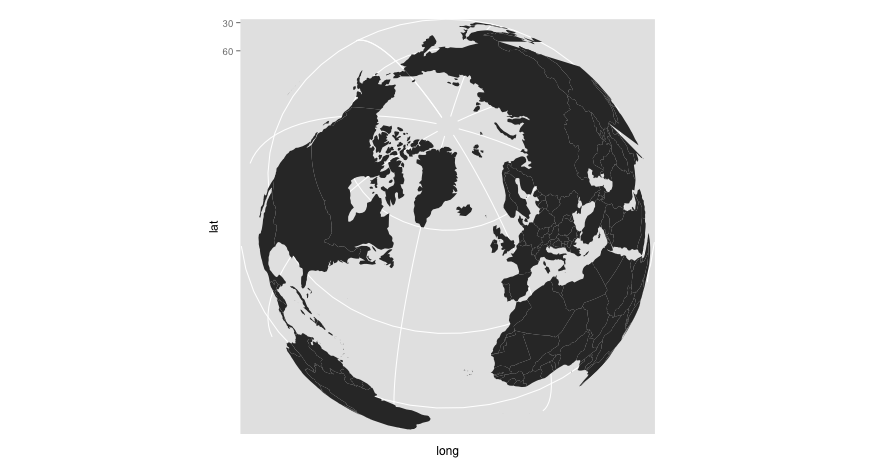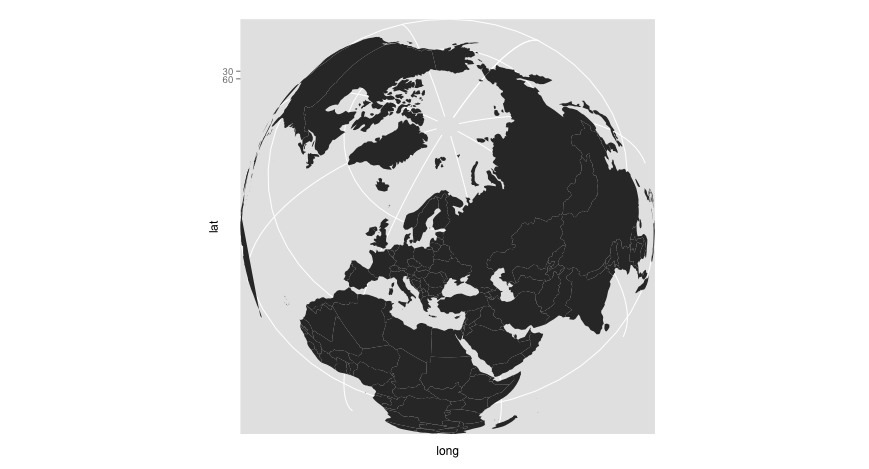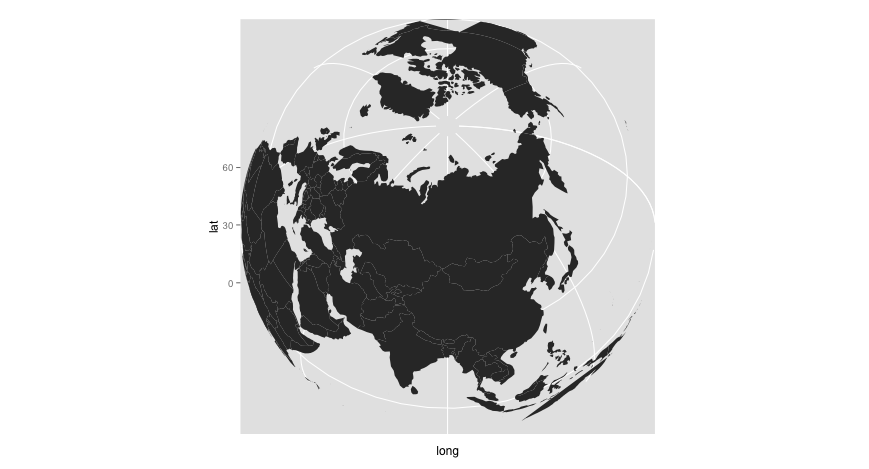Now, we wanted to highlight regions in Canada, Russia and Greenland lying 3000km or less away from the North Pole. This seems a pretty mere task, but it gets kind of arduous when dealing with multiple polygons representing a single country.

We need a function that gives the coordinates of points on a circle given a radius and an origin. The tricky thing here, is that the North Pole latitude is (90^circ), but there is an infinity of coordinates for the longitude, and using the destPoint function from the geosphere package does not work when providing the couple ((0,90)). To get around this issue, I cheated a bit, by adding a really small value to the longitude and latitude of the couple ((0,90)).

# --
# Given the long/lat coordinates of an origin (x) and a radius (radius) in km,
# returns the coordinates of 360 points on the circle of center x and radius radius km.
# --
# x (numeric vector) : coordinates of the origin of the circle
# --
# Creation de 360 points distincts sur le cercle de centre
# x et de rayon radius
resul <- do.call("rbind", lapply(0:360, function(bearing) {
res <- destPoint(p = x, b = bearing, d = radius)
rownames(res) <- NULL
return(data.frame(res))
}))
resul$dist <- radius / 1000 return(resul) } # Let's create two circles of radius 1500 and 3000 km and which center is the North Pole circle.1500 <- distantCircle(x = c(0.0000001,89.9999999), radius = 1500*1000) circle.3000 <- distantCircle(x = c(0.0000001,89.9999999), radius = 3000*1000) circles <- rbind(circle.1500, circle.3000) circles$dist <- factor(circles$dist) rect.3000 <- cbind(long = c(-180,-180,180,180,-180), lat = c(min(circle.3000$lat),90,90,min(circle.3000$lat), min(circle.3000$lat)))
rect.3000.poly <- as(rect.3000, "gpc.poly")


Now, we are going to use the union function from the gpclib package to create a single gpc.poly object for each country we are interested in. It will be easier to deal with such an object. Let’s have a look at the coordinates of Canada:

> head(tail(world.df[world.df$region == "Canada",],10),4) long lat group region 2756 -93.72066 77.63433 Canada.29 Canada 2757 -93.84000 77.52000 Canada.29 Canada 2758 -79.26582 62.15867 Canada.30 Canada 2759 -79.65752 61.63308 Canada.30 Canada  As you can see, there are different groups here, because there are 30 polygons defined to draw the borders of Canada in this data.frame. So, for each of these polygons, we are going to extract the coordinates of the intersection with our circle. The following function gives the gpc.poly object for a country: # -- # From a country name and a data.frame obtained using fortify # returns a polygon of the country # -- # country (string) # map.df (data.frame) : a data.frame obtained with fortify # -- polygonCountry <- function(country, map.df){ country.df <- map.df[map.df$region == country,]
country.poly <- lapply(unique(country.df$group), function(group){ country.group.coords <- country.df[country.df$group == group, c("long", "lat")]
return(as(country.group.coords, "gpc.poly"))
})

country.poly.tot <- country.poly[]
if(length(country.poly)>1){
for(i in 2:length(country.poly)){
country.poly.tot <- gpclib::union(country.poly.tot,  country.poly[[i]])
}
}
return(list(country = country, polygon = country.poly.tot))
}


And here is the function that gives the coordinates of the intersections of the country’s polygons and the North Pole circle of radius 3000 km:

# --
# Returns coordinates of the intersection of a country
# and a polygon
# --
# x (list) : result from polygonCountry()
# poly (gpc.poly) : a polygon
# --
getIntersection <- function(x, poly){
poly.intersect <- gpclib::intersect(x$polygon, poly) # then extract the coordinates of the intersection, if there is one if(length(poly.intersect@pts)>0){# if there is an intersection # We have to be careful, because there might be multiple polygons poly.intersect <- lapply(poly.intersect@pts, function(x) cbind(long = x$x, lat = x$y)) nPoints <- unlist(lapply(poly.intersect, nrow)) group <- NULL for(i in 1:length(nPoints)){ group <- c(group, rep(paste(x$country, i, sep = "."), nPoints[i]))
}
poly.intersect <- do.call("rbind", poly.intersect)
poly.intersect <- data.frame(poly.intersect)
poly.intersect$group <- group poly.intersect$region <- x$country }else{# if there is no intersection poly.intersect <- NA } return(poly.intersect) }  We can apply these functions for Canada, Russia and Greenland. I want the circles to be drawn last so they are not hidden by the polygons. So, I make sure their name in the data.frame begin with a “z”. I think there is a more efficient and beautiful way to accomplish this, but I am not aware of it. If you are, please share your method with me in the comment section below. # Let's bring out parts of Canada, Russia and Greenland 3000km or less away from North Pole countries <- c("Canada", "Russia", "Greenland") countries.big.circle <- lapply(countries, function(aCountry){ getIntersection(x = polygonCountry(country = aCountry, map.df = world.df), poly = rect.3000.poly) }) countries.big.circle <- rbind_all(countries.big.circle) # I add "zcircle" before group name, to ensure it is drawn last when calling ggplot2 countries.big.circle$group <- paste("zcircle", countries.big.circle$group, sep = "") countries.big.circle$region <- paste("zcircle", countries.big.circle$region, sep = "") # Now we combine coordinates of every country and the polygons intersecting the circle # of radius 3000 km and which origin is the North Pole world.df2 <- rbind(world.points[,c("long","lat","group", "region")], countries.big.circle[,c("long","lat","group", "region")])  For ggplot to be able to fill only the polygons of our three countries, let’s add a column in world.df2, where the value is not available for every other country: world.df2$fill <- NA
nomsPays <- NULL
for(country in countries){
world.df2[world.df2$region == country, "fill"] <- country world.df2[world.df2$region == paste("zcircle", country, sep = "") ,"fill"] <- paste("zcircle", country, sep = "")

nomsPays <- c(nomsPays, country, paste("zcircle", country, sep = ""))
}
world.df2$fill <- factor(world.df2$fill, levels=c(nomsPays))


And finally, we can print the map!

worldmap <- ggplot() +
geom_polygon(data = world.df2, aes(x = long, y = lat, group = group, fill = fill)) +
scale_y_continuous(breaks = (-2:2) * 30) +
scale_x_continuous(breaks = (-4:4) * 45)

P <- worldmap + geom_path(data = circles, aes(x = lon, y = lat, group = dist, col = dist), linetype = 2) +
scale_colour_manual("Distance", values = c("1500" = "red", "3000" = "blue"), labels = c("1500" = "1500 km", "3000" = "3000 km")) +
coord_map("ortho", orientation=c(61, -74, 0)) +
scale_fill_manual("",
"Russia" = "#7fc1ff", "zcircleRussia" = "#004e99",
"Greenland" = "#66CC98", "zcircleGreenland" = "#009966"),
na.value = "grey80",
guide = FALSE)

P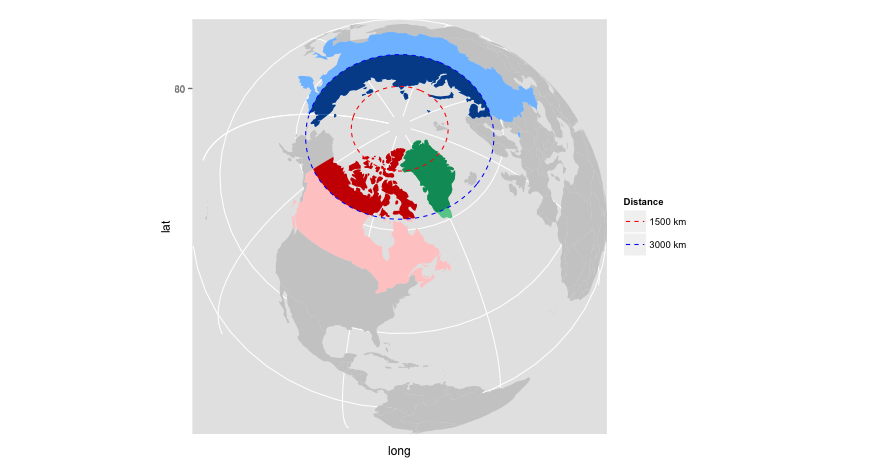Parts of Canada, Russia and Greenland 3000 km or less away from North Pole

Now, if you want to reproduce the animated gif, you can wrap the above code in a function where the argument is the angle:

rotateMap <- function(angle){
worldmap + geom_path(data = circles, aes(x = lon, y = lat, group = dist, col = dist), linetype = 2) +
scale_colour_manual("Distance", values = c("1500" = "red", "3000" = "blue"), labels = c("1500" = "1500 km", "3000" = "3000 km")) +
coord_map("ortho", orientation=c(61, angle, 0)) +
scale_fill_manual("",
"Russia" = "#7fc1ff", "zcircleRussia" = "#004e99",
"Greenland" = "#66CC98", "zcircleGreenland" = "#009966"),
na.value = "grey80",
guide = FALSE)
}

library(animation)

saveGIF({
ani.options(nmax = 360)
for(i in seq(0,360)){
print(rotateMap(i))
}
}, interval = 0.1, outdir="/Users/ewengallic/Documents/Projets/carte_pole", movie.name = "pole_nord.gif")Now, let’s see how to work with gridded data. To illustrate the method, we are going to use some NASA data : GISS Surface Temperature Analysis. There is a link to a txt file for gridded data at the bottom of the page.

First, we need to load the file in the R session.

temp <- read.table("nmaps.txt", skip=1, header = TRUE, na.strings="9999.0000")


If we want to have the same colours and the same breaks on the palette, it is not difficult:

breaks <- c(-5.1, -4, -2, -1, -.5, -.2, .2, .5, 1, 2, 4, 11.1)
lesCouleurs <- cbind(
val = levels(cut(range(breaks), breaks = breaks)),
col = c("#8600FF", "#3F94FE", "#77CAFD", "#99EEFF", "#D9FFD9", "#FFFFFF",
"#FFFF4C", "#FFCC00", "#FF7E00", "#FF0000", "#5E0000")
)
lesCouleurs <- data.frame(lesCouleurs, stringsAsFactors = FALSE)
colnames(lesCouleurs) <- list("val", "col")
# Colours in 8 digits, the last two digits are for transparency
lesCouleurs$col <- paste(lesCouleurs$col,"FF", sep = "")


Then, we can add the interval for the surface temperature anomaly:

temp$interval <- cut(temp$array.i.j, breaks = breaks)


The last step is to create the plot and wrap the code in a function (to change, once again, the angle):

rotate_map <- function(angle = -74){
ggplot() +
geom_tile(data = temp, aes(x = lon, y = lat, fill = intervalle), alpha = 0.8) +
scale_fill_manual("interval", breaks = lesCouleurs$val, values = lesCouleurs$col) +
geom_path(data = world.df, aes(x = long, y = lat, group = group)) +
scale_y_continuous(breaks = (-2:2) * 30) +
scale_x_continuous(breaks = (-4:4) * 45) +
coord_map("ortho", orientation=c(61, angle, 0))
}


Before plotting the map, let’s analyze this code. We want to plot tiles, so we use the geom_tile() function (that makes sense). Data come from temp, where we can find the x and y coordinates. Since we want the tiles to be filled with a colour corresponding to the surface temperature anomaly, we make sure to use the fill argument. And finally, in order to get the same colours as those on the NASA map, we have to change them manually, using the function scale_fill_manual.

rotate_map(1)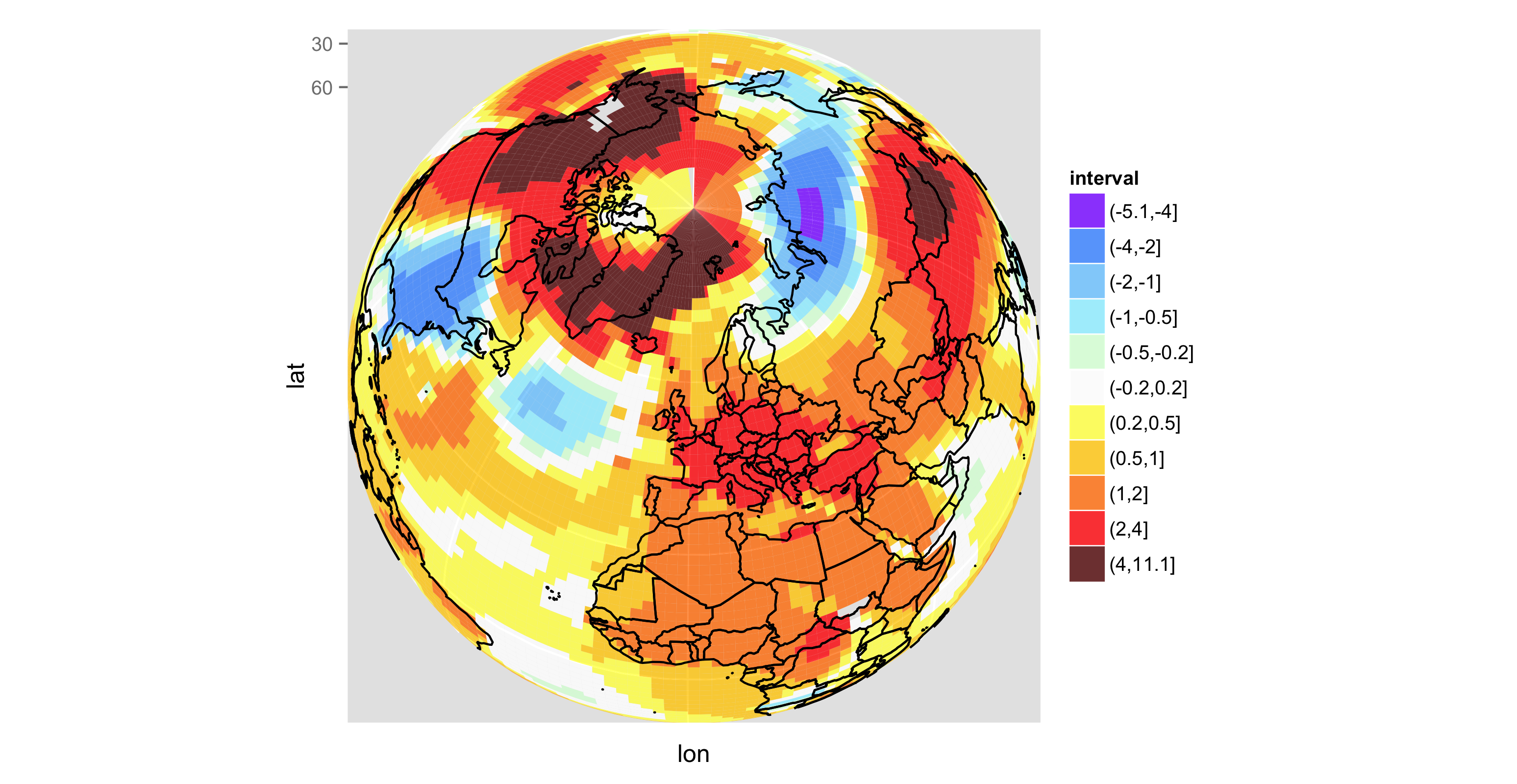Once again, if you want to create an animated version, it is easy, but this time, it takes quite a long time…

library(animation)

saveGIF({
ani.options(nmax = 360)
for(i in seq(0,360)){
print(rotate_map(i))
print(i)
}
}, interval = 0.1, outdir="/Users/ewengallic/Documents/Projets/carte_pole", movie.name = "temp_anomalies.gif")


The result is a huge file (~28 Mb) which can be seen here (I left the legend title in French).

## 6 thoughts on “Maps with R”

1.aurelien says:

alors la chapeau Ewen, c’est spendide.
Tu ne te serais pas faciliter la vie si tu avais utiliser gganimate de D.Robinson pour le GIF?
Bravo encore
aurelien

1.Ewen Gallic says:

Merci Aurélien. Il faut que je mette le nez dans ce package, merci (il n’était pas encore sorti lors de la conception de ces GIFs).

2.Mengjie says:

Hi, Ewen! Thank you for your nice article and codes. I like this! But I have one question about the coordinate for the latitude under the “ortho” map projection. In the map, I found it confused to interpret the latitude numbers on the y axis because it seems not easy to find a corresponding line to each number especially when the figure turns to an animated one (we can see the numbers on the y axis also move). Could you give more hints about that? Thanks!

1.Ewen Gallic says:

Hi Mengjie, I agree with you. It might be a better idea to hide these coordinates, right?

3.Chen says:

Hi Ewen, thank you very much for your nice code and detail explanation on how to plot maps using ggplot2. I appreciate it. I wanted to plot Arctic sea ice. I modified your code to satisfy my purpose. However, I run into a problem.
I can create a sea ice map from these codes: library(ggplot2)
ggplot()+
geom_tile(data =data_1,aes(x = lon, y = lat, fill = seai), alpha = 0.8)

I can also create an Arctic base map based on your code:
ggplot()+
geom_path(data = world.df, aes(x = long, y = lat, group = group)) +
scale_y_continuous(breaks = (-2:2) * 30) +
scale_x_continuous(breaks = (-4:4) * 45) +
coord_map(“ortho”,ylim=c(60,90))

However, if we combine these two together, it takes about 1 hour to create the plot.
ggplot()+
geom_tile(data =data_1,aes(x = lon, y = lat, fill = seai), alpha = 0.8)+
geom_path(data = world.df, aes(x = long, y = lat, group = group)) +
scale_y_continuous(breaks = (-2:2) * 30) +
scale_x_continuous(breaks = (-4:4) * 45) +
coord_map(“ortho”,ylim=c(60,90))

I wanted to ask how long it took for you to create your plot.
Would you please take a moment to check and give me a hint about the reason why it is so slow?

Thank you so much.

Best wishes,
Chen